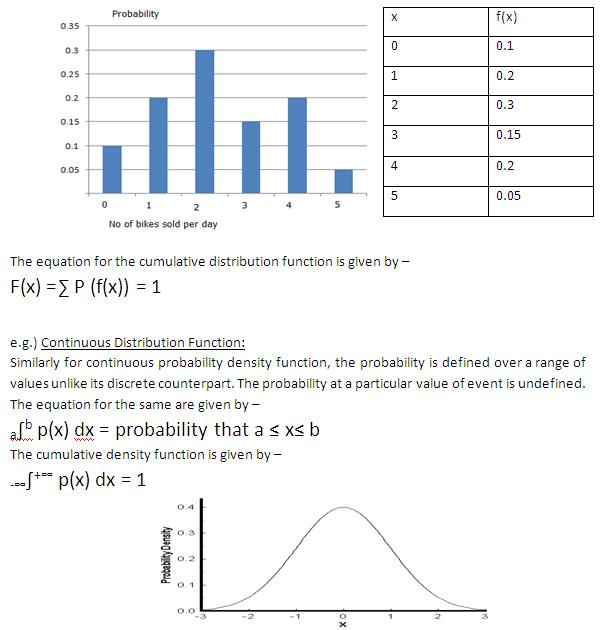Probability Distribution Function

Posted in Statistics, Total Reads: 2625

Definition: Probability Distribution Function

Probability Distribution Function is a function which assigns probability to each of the possible outcomes of a random variable.

Probability Distribution Function for continuous random variable is called Probability Density Function while the one for discrete random variables is called the Probability Mass Function.

The probability distribution function is mainly characterized by cumulative distribution function which represents the summation of probabilities of all possible events.

e.g.)Discrete Distribution Function:

Consider an example of discrete probability distribution function. The figure below shows the probability of selling a specific number of bikes by a dealer per day. The X-axis has  all the possible number of bikes sold and corresponding probabilities are represented in Y-axis.Browse the definition and meaning of more terms similar to Probability Distribution Function. The Management Dictionary covers over 7000 business concepts from 6 categories.

Search & Explore : Management Dictionary

Similar Definitions from same Category: